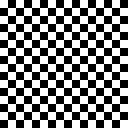## ENTROPY

 Computes the normalized entropy of an image channel-by-channel

 Image And Spectrum Original Image EntropyGray Entropy = 0.125Gray Entropy = 0.873Red Entropy = 0.523204 Green Entropy = 0.52306 Blue Entropy = 0.567637 Average RGB Entropy = 0.537967Red Entropy = 0.905032 Green Entropy = 0.941855 Blue Entropy = 0.870867 Average RGB Entropy = 0.905918Red Entropy = 0.92939 Green Entropy = 0.983668 Blue Entropy = 0.980503 Average RGB Entropy = 0.96452